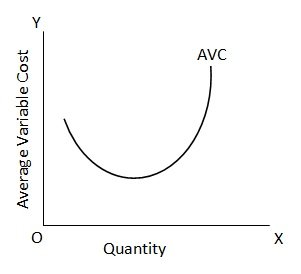Average Variable Cost

# Average Variable Cost Assignment Help

Home / Microeconomics Assignment Help / Average Variable Cost

# Average Variable Cost

Average Variable Cost is equal to total variable cost divided by the quantity produced or AVC = TVC/Q. the average variable cost may be different at different levels of output. In the initial stages of production when TVC increases less than proportionately with increase in output due to the law of increasing returns (or diminishing costs), AVC will be falling. However, beyond a certain output level, TVC will start increasing more than proportionately to the increase in quantity produced and therefore AVC will be rising. This is shown in column 6 where AVC falls from \$ 2 to \$ 1.60 as output increases from 10 units to 50 units, but then continues to rise till it goes \$ 2.50 as output reaches 80 units. Thus, AVC is negatively related to output in some range of output, but this relationship becomes positive because law of diminishing returns leads to eventually increasing average variable cost. Where AVC curve is falling over a certain range of output and thereafter starts rising.### Submit Homework

Submit your homework for a free quote